﻿ 5. How To use « TIMi – StarDust module »? > 5.5. How to create a segmentation? > 5.5.2. The parameters of the K-Means algorithm

# 5.5.2. The parameters of the K-Means algorithm

The K-means algorithm is illustrated in detail in the section 2.2.1.

Here is a brief description of the standard K-means algorithm:

1.We assign randomly one individual to each segment. These “special” individual are named “seeds”. Usually, different “seeds” will give different (but hopefully close) segmentations. Thus, there is no unique segmentation: Depending on the “seeds” you will usually find different segments.

2.We assign all the individual to the nearest segment “center”. To compute which segment is the closest one, we use the “distance-definition” as defined in the previous section.

3.We re-compute the “center” of each segment: it’s the “center of mass” of all the points/ individual inside each each segment.

4.If we have reached the maximum number of iteration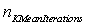then stop. If some individuals have changed from segment, return to step 2, otherwise stop.

To summarize, the objective of the K-means algorithm is to find the center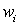of each segment. The “optimal, best centers” are the centers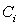that give the lowest “Energy”. The “Energy” is defined this way:... where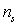is the number of segments (supplied by the user).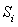is the set of individuals belonging to the ith segment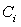is the center of the ith segment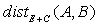is the distance between the individuals A and B, as defined in the previous section

The K-means algorithm tries to find the “best centers” (i.e. the centers that give the lowest energy) but, depending on the “seeds” used, it will only find solutions (i.e. centers) that are more or less close to the “best centers”. This is why, it’s interesting to run the K-means algorithm several times (this number of run is a user-specified parameter named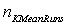) and to select, at the end, the “centers” (i.e. the segmentation) that gave the lowest “Energy”.

Stardust uses a small variation on the standard K-means algorithm named “K-means++” that generates a far better segmentation than the “normal” K-Means. The “K-means++” is the same algorithm as the standard K-means expect that the “seeds” are computed in a slightly different way. The step 1 of the standard K-means algorithm is replaced by:

1.Choose an initial centeruniformly at random amongst our dataset.

2.Choose the next center, selecting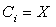with probability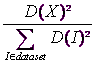...where I is one Individual inside our dataset

D(X) is the shortest distance from the point X to the closest center that we have already chosen

3.Repeat step 2 until we have choose a total of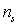centers.

The “K-means++” algorithm has also a strong random component, so it’s good to run it several time (this number of run is a user-specified parameter named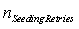) and to select, at the end, the “run” that gave the lowest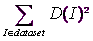value.

The “K-Means” main parameters are defined here (the 5 controls in blue will be described below):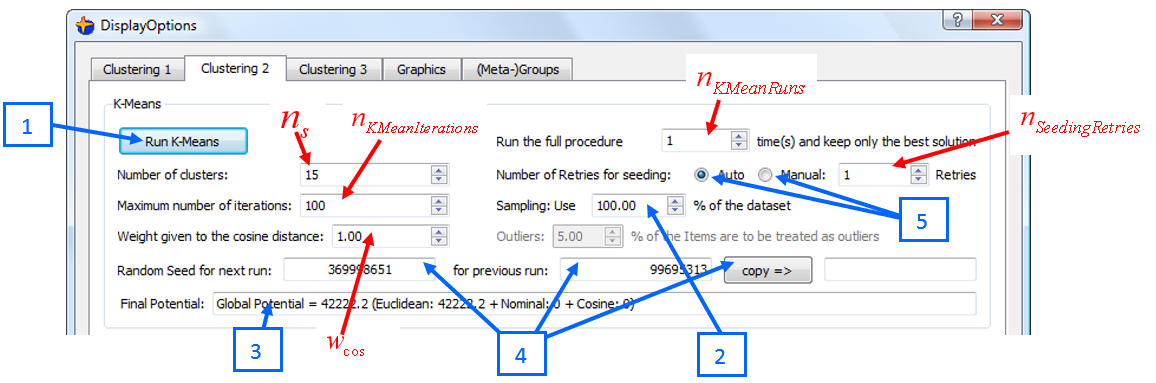Here are some explanations relative to the blue controls in the screenshot here above:

# 5.5.2.1. Control 1: Run K-means

When you click the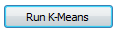button, the K-means algorithm runstimes and then “injects” the best solution found (with the lowest Energy) inside the Ward’s Algorithm. See next section 5.5.3. about the Ward’s Algorithm.

Thebutton is completely equivalent to the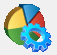button inside the toolbar in the main windows of StarDust.

# 5.5.2.2. Control 2: Sampling

The K-means algorithm can compute for a very long time when the dataset to analyze is very big. To reduce computation time, you can use the “sampling” parameter to reduce the size of the dataset “injected” into the K-means algorithm.

The dataset that will be sampled and “injected” into the K-means algorithm is defined here: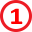In the ideal case, this dataset should not contain any outliers because the K-means algorithm is a little bit sensitive to outliers (this is due to the “squaring effect” of the Euclidean distance).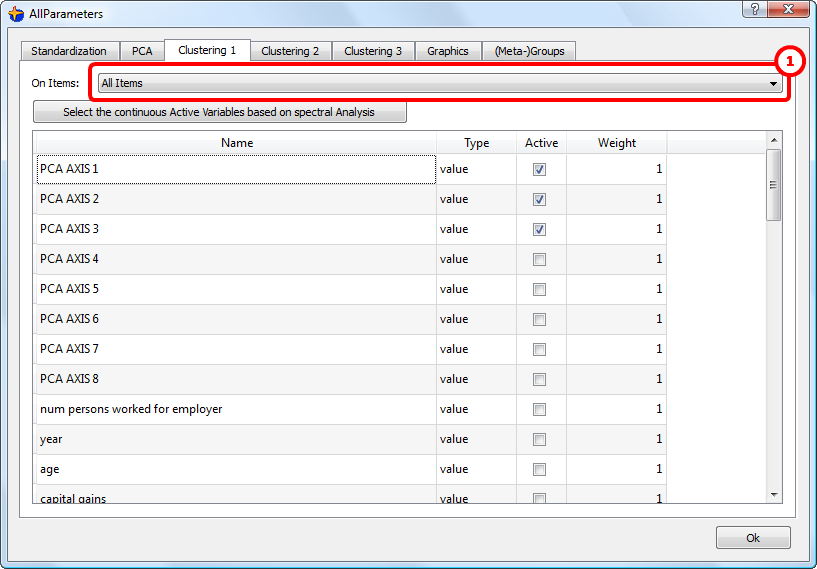# 5.5.2.3. Control 3: Energy

This control displays the “Energy” of the solution found by the K-means algorithm.

To remind you, the “Energy” is defined by:(equation 1)

This can be decomposed in two different ways:

1.When no variables are included inside a “cosine-distance” (the C set is empty)

We have the following “distance-definition”: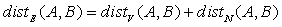(equation 2)

... combining  equations 1 and 2, we find: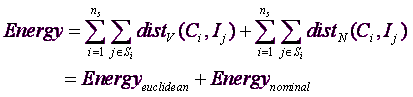... which is displayed here: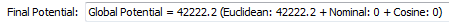2.When some variables are included inside a “cosine-distance” (the C set is non-empty)

We have the following “distance-definition”: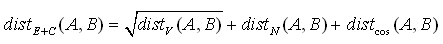(equation 3)

... combining  equations 1 and 3, we find: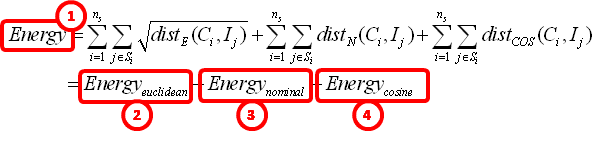... which is displayed here: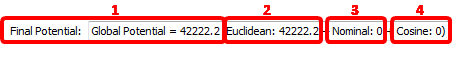This display has several interest points:

1.It’s interesting to look at the “Global Energy” because it’s an estimation of the quality of your segmentation. The lower “Global Energy”, the better the segmentation (at least from a pure mathematical point-of-view). This is a way to “compare” different segmentations and take the best one.

2.It’s interesting to see how the “Global Energy” is distributed amongst the “Euclidean Energy”, “Nominal Energy” and “Cosine Energy” parts, in order to better adjust the,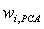and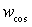parameters. For example, if you see that the largest portion of the “Global Energy” comes from the “Cosine Energy”, you can decrease theparameter.

3.The “Global Energy” is proportional to the number of points inside your dataset. Furthermore, if there are some outliers inside your dataset, then, because of these outliers, the “Global Energy” will have a very high value. When you remove points from your dataset, the “Global Energy” will decrease.  If you remove outlier points from your dataset, then the “Global Energy” decreases substantially. If you continue to remove (non-outlier) points from your dataset, then the “Global Energy” won’t decrease very much. Thus, the “Global Energy” can be used to track if there still exists outliers inside your dataset. The presence of outliers inside your dataset deteriorates the quality of you segmentation and you should avoid them.

4.If you use a “sampling parameter”<100%, then, each time you run the K-means algorithm, you will use a slightly different dataset. If you are lucky enough (i.e. if you set the parameter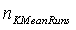high enough), one of these dataset will be composed only by “regular points” (i.e. It won’t contain any outliers). The “Global Energy” of a dataset without any outliers is strongly smaller than the “Global Energy” of a dataset containing outliers. Thus, amongst all the segmentations results available (when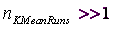), the segmentation based on a dataset containing only “regular points” will always be selected as the final one. Thus, the sampling parameter can be used to “avoid” datasets containing outliers. A strong sampling (50%) will almost certainly remove all outliers but it will also give less information to the K-means algorithm, giving, at the end, a poor segmentation. Some trade-off is to be made. Observing the “Global Energy” allows you to set a good value for the “sampling parameter” and theparameter.

# 5.5.2.4. Control 4: Random seed

The “K-Means++” algorithm is an algorithm with a strong “random” component. Inside Stardust, all the random elements of the “K-means++” are based on a “pseudo-random number generator”. Such kind of generators generates sequences of random number. All the “random decisions” of the “K-means++” algorithm are taken based on the generated random sequence of number. The particularity of “pseudo-random number generators” is that you must initialize them using a first user-supplied number. This number is named “random seed”. The same “random seed” will always produce exactly the same sequence of random number. It means that if you use the same “random seed” number for two different runs of the “K-Means++” algorithm, you will obtain exactly the same segmentation. In other words, the “random seed” number completely defines the segmentation delivered by the “K-Means++” algorithm.

You can “save” good segmentations by saving the corresponding “random seed” number. The “random seed” number that has just been used to produce the current segmentation is visible here:... and you can “save it for later” by clicking here: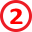You can “restore” good segmentations by, first, entering the corresponding “random seed” number here:... and, second, clicking thebutton.

Thus the “random seed” parameter allows you to quickly “switch” between different segmentations. This is a nice complement to the standard load&save functionalities of StarDust (see section 5.2.2 and 5.2.3 about the load&save segmentation analysis).

# 5.5.2.5. Control 5: retries for seeding

The parameterof the “K-Means++” algorithm is set here: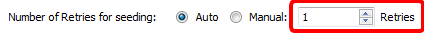In manual mode, the value ofis exactly the one entered by the user inside StarDust.

In “Auto” mode, the value ofis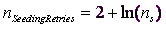(is the number of segments).